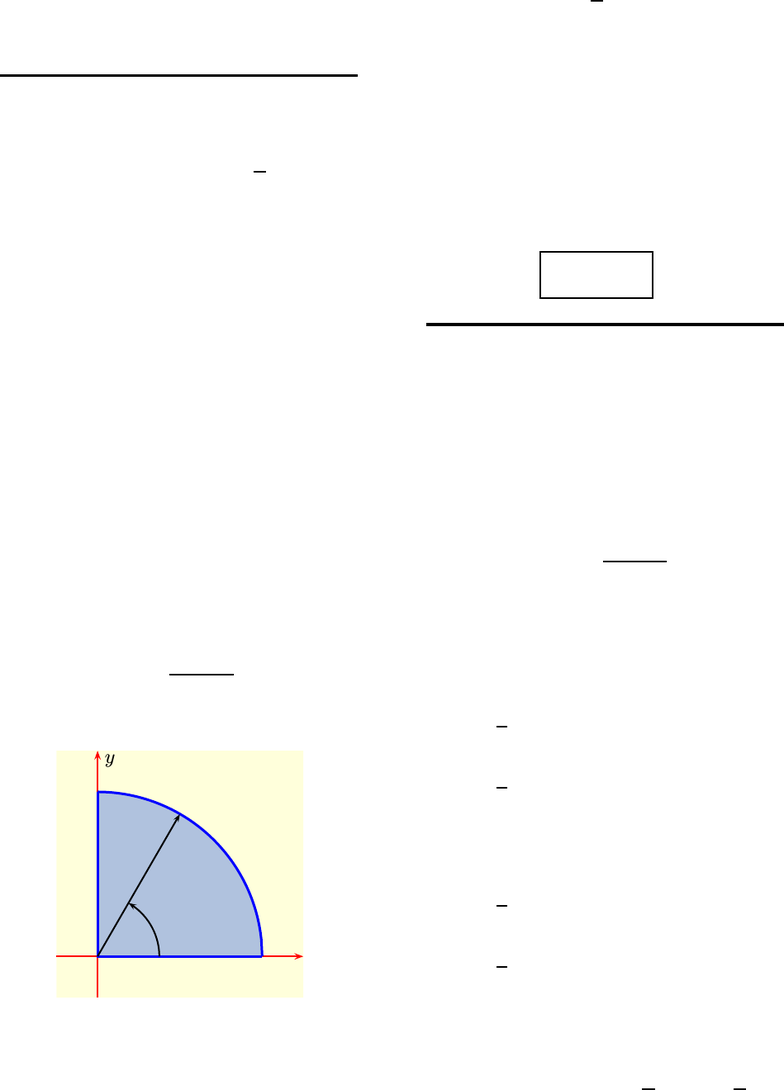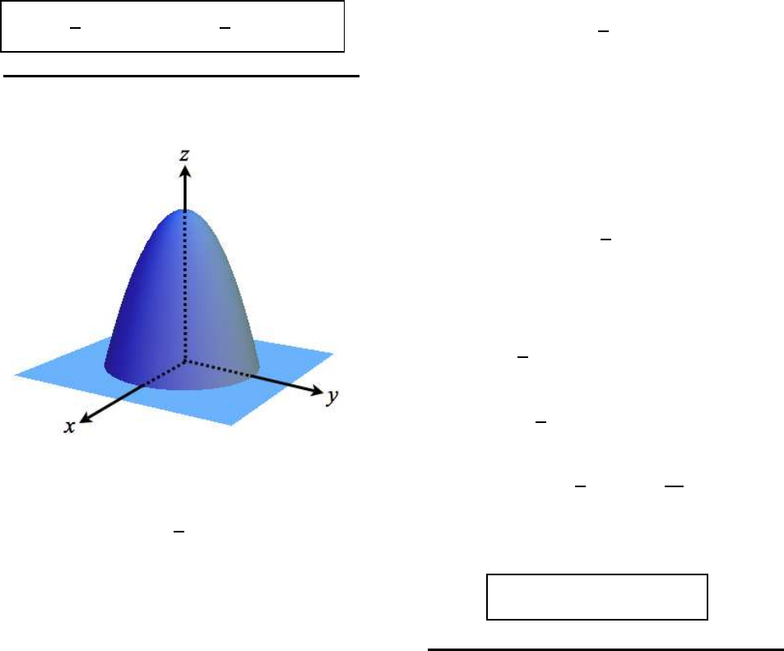# M 408D Chapter Notes - Chapter 15.3: 2 On, Hedeby Stones, The Graphic

34 views7 pages
School
UT-Austin
Department
Mathematics
Course
M 408D
Professoroliver (smo998) – HW 15.3 – rusin – (52440) 1
This print-out should have 10 questions.
Multiple-choice questions may continue on
the next column or page – ﬁnd all choices
001 10.0 points
Evaluate the integral
I=Z ZDn(3π4 tan1y
xodxdy
when Dis the region in the ﬁrst quadrant
inside the circle x2+y2= 16.
1. I=π
2. I= 16 π
3. I= 16 π2
4. I=π2
5. I= 8 π2correct
6. I= 8 π
Explanation:
In Cartesian coordinates the region of inte-
gration is
n(x, y) : 0 yp16 x2,0x4o,
which is the shaded region in
x
y
4
θ
r
On the other hand, in polar coordinates the
region of integration is
n(r, θ) : 0 r4,0θπ/2o,
while
tan1y
x=θ .
Thus in polar coordinates,
I=Z4
0Zπ/2
0
(3π4θ)rdr
=Z4
0h3πθ 2θ2iπ/2
0rdr =π2Z4
0
r dr .
Consequently,
I= 8π2.
002 10.0 points
By changing to polar coordinates evaluate
the integral
I=Z ZR
ex2y2dxdy
when Ris the region in the xy-plane bounded
by the graph of
x=p16 y2
and the y-axis.
1. I=π(1 e16)
2. I=1
4π(1 e16)
3. I=1
2π(1 e16)correct
4. I=π(1 e4)
5. I=1
4π(1 e4)
6. I=1
2π(1 e4)
Explanation:
In polar cooordinates, Ris the set
n(r, θ) : 0 r4,π
2θπ
2o,
Unlock document

This preview shows pages 1-2 of the document.
Unlock all 7 pages and 3 million more documents.oliver (smo998) – HW 15.3 – rusin – (52440) 2
while
I=Z ZR
er2(rdr)
=Z ZR
rer2drdθ ,
since x2+y2=r2. But then
I=Z4
0Zπ/2
π/2
rer2dr
=πZ4
0
rer2dr .
The presence of the term rnow allows this last
integral to be evaluated by the subsitution
u=r2. For then
I=1
2πheui16
0=1
2π(1 e16).
003 10.0 points
The solid shown in
is bounded by the paraboloid
z= 4 1
4(x2+y2)
and the xy-plane. Find the volume of this
solid.
1. volume = 32
2. volume = 8 π
3. volume = 16 π
4. volume = 16
5. volume = 32 πcorrect
6. volume = 8
Explanation:
The paraboloid intersects the xy-plane
when z= 0, i.e., when
x2+y216 = 0 .
Thus the solid lies below the graph of
z= 4 1
4(x2+y2)
and above the disk
D=n(x, y) : x2+y216 o,
so its volume is given by the integral
V=Z ZD41
4(x2+y2)dxdy .
In polar coordinates this becomes
V=1
4Z4
0Z2π
0
r(16 r2)dr
=1
2πZ4
0
(16rr3)dr
=1
2πh8r2r4
4i4
0.
Consequently,
volume = V= 32 π .
004 10.0 points
The solid shown in
Unlock document

This preview shows pages 1-2 of the document.
Unlock all 7 pages and 3 million more documents.